Previous: 4.6 Speedup Algorithms Up: 4.6 Speedup Algorithms Next: 4.6.2 Trajectory-Reuse Method

## 4.6.1 Trajectory-Split Method

The impurity distribution which is calculated when simulating ion implantation is proportional to the probability distribution that an implanted ion reaches a certain area of the simulation domain. Regions where the impurity concentration is two orders lower than the maximal concentration level in the simulation domain, are reached just by one of hundred implanted ions. The situation is even worse at lower concentration regions. A reasonable simulation result has to represent three to five orders of the impurity concentration, where at least some particles have to reach the zones of the lowest concentration to ensure an acceptable statistical accuracy, because from the presence of just one particle no meaningful concentration information can be derived.

It is obvious that at least several ten thousands of ion trajectories have to be simulated to fulfill this requirement where most of these trajectories end up within regions of a high impurity concentration and therefore do not significantly contribute to an improvement of the simulation result. To reduce the number of such irrelevant trajectories the Trajectory-Split method is applied (, , ).

The fundamental idea is to increase the number of ion trajectories that end up in regions of low impurity concentration by splitting a particle trajectory in case the particle moves from a region of higher concentration to a region of lower concentration as indicated in Fig. 4.17.Splitting means that originating from one particle several new particles are created with the same physical properties as the original particle, but with a reduced weighting factor. As mentioned in Sec. 4.5.2 all simulation results generated during the simulation, like a change in the number of vacancies, interstitials or impurities are multiplied with the weighting factor before they are stored. While for the molecular method a weighting factor larger than one is used to account for statistical identical particles of the same atom species in a molecule, the weighting factor is reduced below one by the Trajectory-Split method to conserve the total implantation dose. According to  it is most efficient to split one particle into two new particles and to apply the splitting process recursively until a maximum split depth of eightso that the minimal weight of a particle is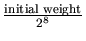.

Various criteria are applied to decide if a particle can be split.

• Energy Criterion:
The splitting of a particle is forbidden if the ratio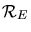of the actual particle energy and the initial particle energy is above a minimum energy threshold level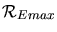to avoid too many split points.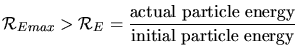(4.9)

A particle species and energy dependent value foris proposed in  for the particle species boron, phosphorus, silicon and arsenic as shown in Fig. 4.18, while a default value of 0.4 is used for all other particle species.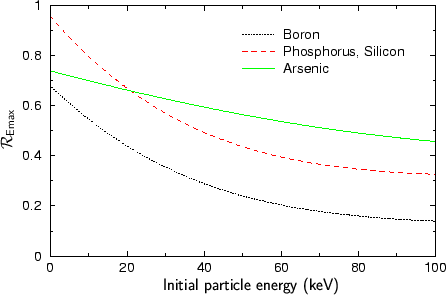• Trajectory Length Criterion:
The splitting of a particle is forbidden, if the ratio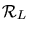of the length of the trajectory up to the potential split point, and the average trajectory length, is shorter than the minimal length ratio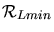. The average trajectory length is accurately determined during the simulation, while at the beginning an estimated average length equivalent to an estimated projected range is used.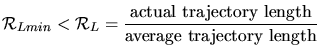(4.10)

As for the energy ratio a particle species and energy dependent value foris proposed in  for the particle species boron, phosphorus, silicon and arsenic as shown in Fig. 4.19.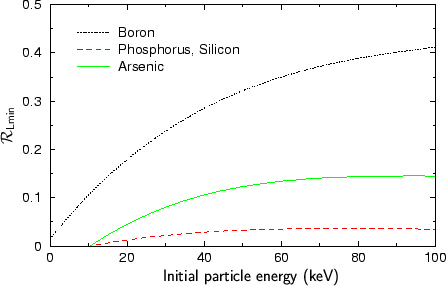The default valuefor all other species is 0.1. Due to this criterion mainly particles in the channeling region are split.
• Trajectory Density Gradient Criterion:
In the ideal case the aim of the Trajectory-Split method is to reach an equivalent number of trajectory end points in all histogram boxes independent of the impurity concentration described by a certain histogram box. Therefore the trajectory end points are stored in an individual histogram, one for each particle species. While the weighting factor is considered when storing data in the normal species histograms this factor is neglected when storing trajectory endpoints. A particle trajectory is only split if the particle moves from a region with a higher number of trajectory endpoints to a region with a lower number of trajectory endpoints. This is checked by defining several levels of trajectory numbers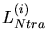. By default 10 levels are defined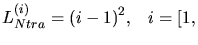number of levels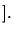(4.11)

If a particle moves to a region corresponding to a lower level the trajectory is split if all other requirements for splitting are met.
• Energy Loss Criterion
A particle must loose a certain amount of energy between two trajectory split points. According to  it significantly improves the efficiency of the Trajectory-Split method if this minimal energy loss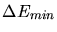is 6.6 % of the minimal energy for splitting according to the energy criterion.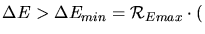initial particle energy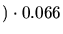(4.12)

To guarantee that two split trajectories are not identical after a split point there must at least one effect in the calculation of a particle trajectory which randomly influence the motion of the particle. If this is not the case two trajectory split branches are identical because the initial conditions at the split point are identical. In that case the Trajectory-Split method does not yield any performance gain. It would even significantly decrease the performance because because identical trajectory would be calculated several times.

In crystalline materials the vibration of the lattice atom guarantees this randomness while in amorphous materials the collision partners are placed randomly. Nevertheless, the Trajectory-Split method is only applied within crystalline materials, because its original intention was to improve the statistical accuracy in the channelling regions.

The effect of the Trajectory-Split method is that one implanted ion delivers several effective ions which share the initial part of the trajectory. Therefore less initial ions have to be used to generate a simulation result with the same statistical accuracy. Due to the sharing of parts of the trajectories the average calculation time for a trajectory of one effective ion is reduced. Worth mentioning is that in case of applying the Follow-Each-Recoil method the recoiled atoms are not split, because the number of recoils is already significantly higher than the number of implanted particles.

#### Footnotes

... eightInstead of using the default value for the split depth a value can be specified by the command-line parameter nbSplitLevel.
... speciesThe value ofcan also be set by the command-line parameter relativeSplitEnergy.
... valueIt can be overridden by the command-line parameter relativeSplitLength.
... definedThe number of levels can be defined by the command-line parameter nbConcentrationLevel.Previous: 4.6 Speedup Algorithms Up: 4.6 Speedup Algorithms Next: 4.6.2 Trajectory-Reuse Method

A. Hoessiger: Simulation of Ion Implantation for ULSI Technology